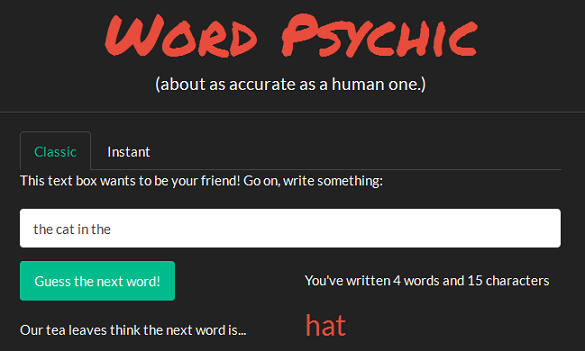# Word Psychic

Melissa Tan
April 2015

Made for Coursera data science specialization, Swiftkey capstone project.

### App descriptionThe app lets the user type in a phrase. It predicts the most likely next word, based on frequently occurring phrases (n-grams).

I used 2-grams to 5-grams, drawn from ~12,000 lines of English from blogs, news articles and Twitter.

I cleaned the text to remove things like punctuation (except apostrophes). I also replaced all words that appeared only once with a placeholder, unk. These could be typos or words like “zzzzzz”.

### App function and instructions

How the app works:

1. User inputs a phrase (as many words as she wants)
2. App looks at the last 1-4 words in phrase
3. App checks the database for phrases that match
4. App gathers all the possible predicted words and displays the most likely one

How to use the app:

• Classic mode: Type into the text field, and click the button to see the predicted next word.
• Instant mode: Type into the text field, and wait. The app automatically displays the predicted next word.

### Behind the scenes: Algorithm used

The algorithm used is Stupid Backoff, described in Brants et al, 2007 (link, see section 4). I chose this because it is inexpensive and loads fast, while performing nearly as well as Kneser-Ney smoothing – which is quite good.

The algo gives each candidate word a score, based on its n-gram frequency. Mathematically, let $$Score = \begin{cases} \frac {freq(w_i)_{n=k+1}} {freq(w_{i-k}^{i-1})_{n=k+1}} & \text{if } freq(w_{i-k}^i)_{n=k+1} > 0 \\ \alpha \frac {freq(w_i)_{n=k}} {freq(w_{i-(k-1)}^{i-1})_{n=k}} & \text{otherwise} \end{cases}$$

Hypothetical example: user types cat in the. One possible next word is hat. We look in the 4-gram first (n = 4). The score for hat is given by: $Score(\text{"hat"}) = \begin{cases} \frac {freq(\text{"hat"})_{n=4}} {freq(\text{"cat in the"})_{n=4}} & \text{if } freq(\text{"cat in the hat"})_{n=4} > 0 \\ \alpha \frac {freq(\text{"hat"})_{n=3}} {freq(\text{"in the"})_{n=3}} & \text{otherwise} \end{cases}$

Note that the score depends on the relative frequency, which is also the Maximum Likelihood Estimate (MLE), in this case.

### Algorithm walk-through example

User input: "The CAT, in the ?". What the algo does is:

• Cleans up and standardizes the input, turning it into: "the cat in the".

• Check 5-gram data for all occurrences of the cat in the *, where * denotes any word. Similarly, check 4-gram data for cat in the *, check 3-gram data for in the *, check 2-gram data for the *. Make a list of all the * candidate words.

• For each word, find maximum likelihood estimate (MLE) in the corresponding n-gram, compute overall score (set $$\alpha$$ = 0.4), and produce a score table. Below are the first 3 rows of an example score table, which show that hat followed 100% of the the cat in the instances in the 5-gram.

nextword n5.MLE n4.MLE n3.MLE n2.MLE score
hat 100 100 0 0 100.0
unk 0 0 3 4 0.5
first 0 0 2 1 0.3
• Remove any unk rows. (since unk was a placeholder for words that only appeared once)

• Output the word with the top score. If there are multiple words with the same top score, randomly pick one. If user turns on safe mode and the output word is a profanity, censor the output.